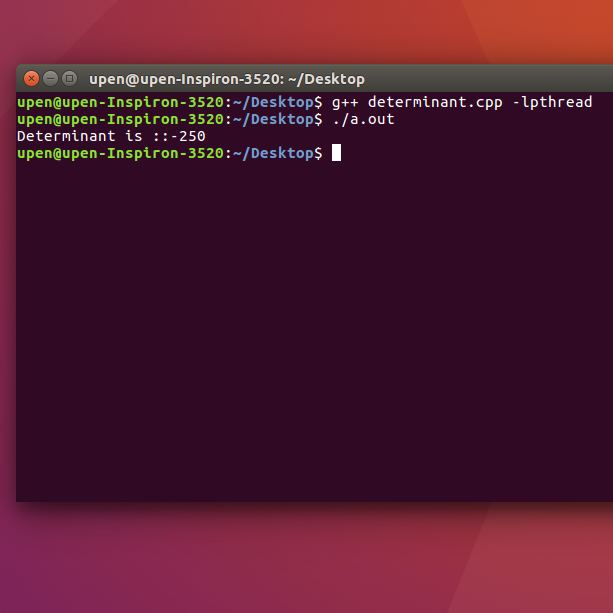Skip to content
Related Articles
Determinant of N x N matrix using multi-threading
• Last Updated : 20 Mar, 2018

Given a matrix of N x N, task is to find the determinant of the matrix using multi-threading.

Examples :

```Input : mat = {{0, 4, 0, -3},
{1, 1, 5, 2},
{1, -2, 0, 6},
{ 3, 0, 0, 1}}
Output : -250

Input :  mat = {{1, 0, 2, -1},
{3, 0, 0, 5},
{2, 1, 4, -3},
{1, 0, 5, 0}}
Output: 30
```

Approach :
It is known that finding the determinant of a matrix can be very costly in the amount of time it takes. So, computing things parallely may lead to a comparatively less costly program. A code can be parallelized using threads, which is light weight process and divides a single flow program into a programs with multiple flows. As for finding the determinant of a matrix, first find the determinant of the submatrices of the matrix, distribute the task of finding the determinant of submatrices to the threads, and these threads will run parallelly resulting in less time execution time than the sequential method.

Note :The code is Linux specific.

## Recommended: Please try your approach on {IDE} first, before moving on to the solution.

 `// CPP program for finding determinant matrix``// with parallalizing the code``#include ``#include ``#include ``#define size 4`` ` `using` `namespace` `std;`` ` `// matrix whoe's determinant is required``int` `mat[][size] = { { 0, 4, 0, -3 },``                    ``{ 1, 1, 5, 2 },``                    ``{ 1, -2, 0, 6 },``                    ``{ 3, 0, 0, 1 } };``int` `det[size];`` ` `// declaring variable for storing thread id``pthread_t ``thread``[size];`` ` `// function for finding determinant``int` `determinant(vector > mat2, ``int` `s)``{`` ` `    ``if` `(s == 2) {``         ` `        ``// if size of matrix is 2X2``        ``// then returning the determinant``        ``return` `mat2 * mat2 - ``               ``mat2 * mat2;``    ``}``    ``else` `{``         ` `        ``// else dividing the matrix in smaller part.``        ``vector > mat1(s - 1), ``                      ``mat3(s - 1), mat4(s - 1);``        ``int` `k, l, m, i, j;``         ` `        ``for` `(i = 0; i < s - 1; i++) {``            ``mat1[i] = vector<``int``>(s - 1);``            ``mat3[i] = vector<``int``>(s - 1);``            ``mat4[i] = vector<``int``>(s - 1);``        ``}``         ` `        ``for` `(i = 1; i < s; i++) {``            ``k = 0;``            ``l = 0;``            ``m = 0;``            ``for` `(j = 0; j < s; j++) {``                ``if` `(j != 0) {``                    ``mat1[i - 1][k] = mat2[i][j];``                    ``k++;``                ``}``                ``if` `(j != 1) {``                    ``mat3[i - 1][l] = mat2[i][j];``                    ``l++;``                ``}``                ``if` `(j != 2) {``                    ``mat4[i - 1][m] = mat2[i][j];``                    ``m++;``                ``}``            ``}``        ``}`` ` `        ``return` `mat2 * determinant(mat1, s - 1) - ``               ``mat2 * determinant(mat3, s - 1) + ``               ``mat2 * determinant(mat4, s - 1);``    ``}``}`` ` `// function for finding determinant using first row``// with each element of row a thread is associated.``void``* createTd(``void``* arg)``{``    ``int` `*ar = (``int` `*)arg, i, j, k;``    ``vector > mat2(size - 1);`` ` `    ``for` `(i = 0; i < size - 1; i++)``        ``mat2[i] = vector<``int``>(size - 1);``         ` `    ``// extracting the matrix smaller by size one.``    ``// for finding the determinant.``    ``for` `(i = 1; i < size; i++) {``        ``k = 0;``        ``for` `(j = 0; j < size; j++) {``            ``if` `(j != (*ar)) {``                ``mat2[i - 1][k] = mat[i][j];``                ``k++;``            ``}``        ``}``    ``}``     ` `    ``// calling determinant function``    ``det[*ar] = det[*ar] * determinant(mat2, size - 1);``}`` ` `// driver function``int` `main()``{``    ``int` `i, j, detfin = 0;``    ``int` `p[size];``     ` `    ``// storing the first row in a array``    ``// for later multipying with the determinant``    ``// of smaller matrix``    ``for` `(i = 0; i < size; i++)``        ``det[i] = mat[i];``         ` `    ``// creating thread``    ``for` `(i = 0; i < size; i++) {``        ``p[i] = i;``        ``pthread_create(&``thread``[i], NULL, &createTd, (``void``*)&p[i]);``    ``}``     ` `    ``// waiting for all the threads to join``    ``pthread_join(``thread``, NULL);``    ``pthread_join(``thread``, NULL);``    ``pthread_join(``thread``, NULL);``    ``pthread_join(``thread``, NULL);``    ``for` `(i = 0; i < size; i++) {``        ``if` `(i % 2 == 0)``            ``detfin += det[i];``        ``else``            ``detfin -= det[i];``    ``}``     ` `    ``cout << detfin << endl;``     ` `    ``return` `0;``}`

Output:Want to learn from the best curated videos and practice problems, check out the C++ Foundation Course for Basic to Advanced C++ and C++ STL Course for foundation plus STL.  To complete your preparation from learning a language to DS Algo and many more,  please refer Complete Interview Preparation Course.

My Personal Notes arrow_drop_up